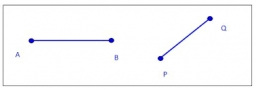# Divide 27883

Divide the line AB 8 cm long in a ratio of 2: 5

a =  2.2857 cm
b =  5.7143 cm

### Step-by-step explanation:Did you find an error or inaccuracy? Feel free to write us. Thank you!

Tips for related online calculators
Need help calculating sum, simplifying, or multiplying fractions? Try our fraction calculator.
Check out our ratio calculator.
Do you want to convert length units?